Ch 6. Entropy Multimedia Engineering Thermodynamics Entropy Tds Relations EntropyChange IsentropicProcess IsentropicEfficiency EntropyBalance (1) EntropyBalance (2) ReversibleWork
 Chapter 1. Basics 2. Pure Substances 3. First Law 4. Energy Analysis 5. Second Law 6. Entropy 7. Exergy Analysis 8. Gas Power Cyc 9. Brayton Cycle 10. Rankine Cycle Appendix Basic Math Units Thermo Tables Search eBooks Dynamics Fluids Math Mechanics Statics Thermodynamics Author(s): Meirong Huang Kurt Gramoll ©Kurt GramollTHERMODYNAMICS - THEORY

Clausius Inequality

The second law of thermodynamics is developed for systems undergoing cycles while exchanging heat with two reservoirs. One corollary of the second law is known as the Clausius inequality, which states that the cyclic integral of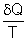is always less than or equal to zero. That is,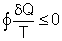where
delta;Q = differential heat transfer at the system
boundary during a cycle
T = absolute temperature at the
boundary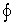= integration over the entire cycle

The Clausius inequality is valid for all cycles, reversible or irreversible.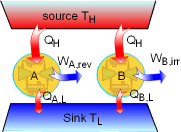Demonstration of the
Clausius Inequality

To demonstrate the Clausius inequality, consider two heat engines that work between the same two reservoirs. Heat engine A is an reversible heat engine and heat engine B is an irreversible one. Both of these two engines absorb heat QH from a heat source, which has a temperature of TH and reject heat to a heat sink at temperature TL. Applying the first law to both of the engines gives,

WA,rev = QH - QA,L

WB,irr = QH - QB,L

Since a reversible engine has the highest efficiency of any heat engines working between the same source and sink, a reversible heat engine produces more work than an irreversible heat engine for the same heat input QH.

WA,rev = QH - QA,L > WB,irr = QH - QB,L

QA,L < QB,L

Since the temperature TH is constant during the heat transfer QH, and the temperature TL is constant during the heat transfer QA,L and QB,L, the cyclic integral offor both heat engines is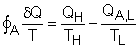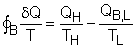Comparing the above two equations with QA,L < QB,L yields,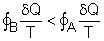(1)

A reversible heat engine holds the following relation: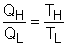Therefore, the cyclic integral offor a reversible engine is zero.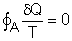Combining the above equation with equation (1) gives,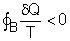To summarize the above analysis, the Clausius inequality states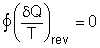for internally reversible cycles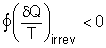for irreversible cycles

Entropy

From the discussion above, , the Clausius inequality states, for an internally reversible cycleThe cyclic integral of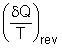is zero. Therefore,is a property. Clausius in 1865 named this new thermodynamic property entropy. The definition is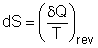The entropy change for process1-2 can be determined as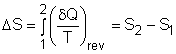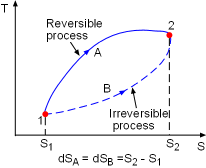The Entropy Change between
two Specified States are the
Same Regardless the
Process Is Reversible or Not

Note that the above definition actually defines the change of entropy, instead of entropy itself. Because entropy is a property, the entropy change between two specified states is the same whether the process is reversible or not.

The previous discussion only means that entropy is a useful property in the second law analysis of an engineering device. But what is entropy? Entropy can be viewed as a measure of molecular disorder. In other words, entropy is the amount of disorder in a system. A pure substance at absolute zero temperature is in perfect order, and its entropy is zero. This is the third law of thermodynamics.

 The entropy of a pure crystallite substance at absolute zero is zero.

If the substance is not a pure crystal like substance, its entropy at absolute zero is not zero.

When the molecular disorder is produced, the ability of doing work is reduced.

The Increase of Entropy Principle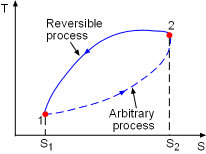Cycle 1-2-1

Consider a cycle as described in the diagram at the left. Peocess1-2 is an arbitrary (reversible or irreversible) process, Process 2-1 is an internally reversible process. From the Clausius inequality,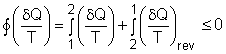or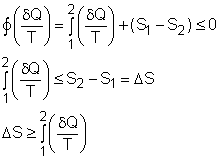where the equality holds for internally reversible process and inequality holds for irreversible process.

For an isolated system, the heat transfer at the system boundary is zero. The above equation becomes

ΔSisolated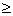0

This equation is known as the increase of entropy principle. It states

 The entropy of an isolated system during a process always increases, or remains constant if the process is reversible.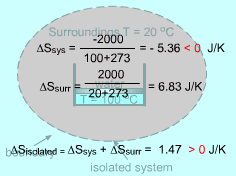Negative Entropy Change of a System

Entropy is an extensive property, and thus the total entropy of a system is equal to the sum of the entropies of all parts of the system. The combination of a system and its surroundings is an isolated system. For this case, the increase of entropy principle is

ΔSisolated = ΔSsys + ΔSsurr0

The entropy change of a system can be negative. But the entropy change of an isolated system formed by this system and its surroundings can not be negative.

The second law of thermodynamics states that processes can occur only in a certain direction. But which direction? The increase of entropy principle answers this question: a process must proceed in the direction that complies with the increase of entropy principle. A process that violates this principle is impossible.